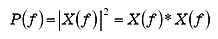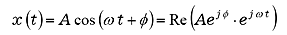FFT analyzer FAQWhat is power spectrum?The power spectrum is obtained when the time signal x (t) is divided into fixed frequency band (frequency resolution Δ f) by processing the FFT analysis, and represents into a graph with the frequency in the vertical axis and signal strength in the horizontal axis.

The FFT analyzer calculates as the following expression (1). When the fourier spectrum of the signal x (t) is X (f), the power spectrum P (f) can be calculated in the following expression (1);(*shows the complex conjugate value) (1)

From the expression (1), the followings can be said for the power spectrum;

 The phase information of the initial signal x (t) will be lost.
 Having the square dimension of the amplitude which means when the physical unit of the signal x (t) is EU (V), it will be EU2 (V2).

For example, if the input signal is x (t)in the expression (2), the power spectrum is A2/2(=(A/√2)2 which is the square of an effective value.(2)

Moreover, the power spectrum corresponds to the square value of the signal amplitude, and we call the square root of this value as a linear spectrum (or amplitude spectrum). This shows that the value is effective value (RMS) of each frequency bandwidth. The linear spectrum of x (t) in the expression (2) is 0.707 A (0.707=1/√2).

Revised:(2009/11/16)